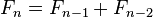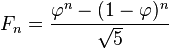# Fibonacci number facts for kids

Kids Encyclopedia FactsA Fibonacci spiral created by drawing a line through the squares in the Fibonacci tiling; this one uses squares of sizes 1, 1, 2, 3, 5, 8, 13, 21, and 34; see Golden spiral

The Fibonacci numbers are a sequence of numbers in mathematics named after Leonardo of Pisa, known as Fibonacci. Fibonacci wrote a book in 1202, called Liber Abaci ("Book of Calculation"), which introduced the number pattern to Western European mathematics, although mathematicians in India already knew about it.

The first number of the pattern is 0, the second number is 1, and each number after that is equal to adding the two numbers right before it together. For example 0+1=1 and 3+5=8. This sequence goes on forever.

 F0 F1 F2 F3 F4 F5 F6 F7 F8 F9 F10 F11 F12 F13 F14 F15 F16 F17 F18 F19 F20 0 1 1 2 3 5 8 13 21 34 55 89 144 233 377 610 987 1597 2584 4181 6765

This can be written as a recurrence relation,$F_{n} = F_{n-1} + F_{n-2}$

For this to make sense, at least two starting points need to be given. Here,$F_{0} = 0$ and$F_1 = 1$.

## Fibonacci numbers in nature

Fibonacci numbers are related to the golden ratio, which shows up in many places in buildings and in nature. Some examples are the pattern of leaves on a stem, the parts of a pineapple, the flowering of artichoke, the uncurling of a fern and the arrangement of a pine cone. The Fibonacci numbers are also found in the family tree of honeybees.

## Binet's Formula

The nth Fibonacci number can be written in terms of the golden ratio. This avoids having to use recursion to calculate Fibonacci numbers, which can take a computer a long time to do.$F_n = \frac{\varphi^n - (1-\varphi)^n}{\sqrt{5}}$

Where$\varphi = \frac{1+\sqrt{5}}{2}$, the golden ratio.

## Images for kidsFibonacci number Facts for Kids. Kiddle Encyclopedia.